# Classification using Support Vector Machine Implemented in R¶

This post demonstrated how classification can be done using Support Vector Machine (SVM). SVM is a supervised learning algorithm used to classify the training data set by finding an optimal hyperplane that separates the class labels. The purposes of training, validating and test datasets in the course of model development are discussed. The dataset is obtained from a Machine Learning online course material offered by Dr Andrew Ng through Coursera. We have implemented SVM in the class using Octave software. Here I show how to implement it using R as well as the importance of the three datasets.

Before implementing SVM in R, I briefly explain the theories behind hypothesis function, cost function and decision boundary. Then I will show step by step how to implement SVM using R.

Hypothesis Function: The hypothesis function $(h_\theta(x))$ is the function that is used to estimate the output (y) from the input data (X). The hypothesis function for SVM is: $$h_\theta(x)= \frac{1}{1+e^{-z}}$$

$$z=\theta^Tx$$

Where $\theta$ is a vector of weight parameters that are estimated from objective function (see below). $\theta^T$ stands for the transpose of $\theta$. X (capital) is the matrix of predictor variables or features, x (small) is a vector of the value of predictor variables of an observation. Unlike logistic regression, $h_\theta(x)$ is not a probability that the output is 1 or 0. Rather, it outputs either 0 or 1. $$h_\theta(x)=\{\begin{array}{rcl}1&\mbox{if}&\theta^Tx\geq0\\0 & \mbox{otherwise}\end{array}$$

Cost Function for SVM: Cost function measures the accuracy of the hypothesis function. This means that it measures how much the predicted output deviates from the actual value. The parameters $(\theta)$ are estimated in such a way that the cost function is minimized. The cost function for SVM with regularization is: $$J(\theta)=C\sum_{i=1}^m[-y^{(i)}(\log(h_\theta(x^{(i)})))-(1-y^{(i)})\log{(1-h_\theta(x^{(i)})})]+ \frac{1}{2}\sum_{j=1}^n\theta_j^2$$

$$J(\theta)=C\sum_{i=1}^m[y^{(i)}(-\log{\frac{1}{1+e^{-\theta^Tx^{(i)}}}})+ (1-y^{(i)})(-\log(1-{\frac{1}{1+e^{-\theta^Tx^{(i)}}}}))] + \frac{1}{2}\sum_{j=1}^n\theta_j^2$$$$J(\theta)=C\sum_{i=1}^m[y^{(i)}(cost_1(\theta^Tx^{(i)}))+ (1-y^{(i)})(cost_0(\theta^Tx^{(i)}))] + \frac{1}{2}\sum_{j=1}^n\theta_j^2$$

Where $J(\theta)$ is the cost function we want to minimize, $y^{(i)}$ is the actual class label of the $i^{th}$ observation, C is regularization parameter that is used to control under fitting or overfitting, $cost_1(\theta^Tx^{(i)})$ is the cost of classifying when y=1, and $cost_0(\theta^Tx^{(i)})$ is the cost of classifying when y=0.

Decision Boundary: The decision boundary or hyperplane in SVM is created in such a way that the distances from the boundary to its closest data points are as large as possible. Those data points closest to the decision boundary are called support vectors and influences optimality. The distance from the boundary to the support vectors is called margin. SVM maximizes the margin around the separating hyperplane. The regularization parameter (C) has to be very large in order to achieve large margin. When C is large, the term $\sum_{i=1}^m[y^{(i)}(cost_1(\theta^Tx^{(i)}))+ (1-y^{(i)})(cost_0(\theta^Tx^{(i)}))]$ from the cost function has to be close to zero to have minimum cost. Thus, the cost function reduces to : $$J(\theta)=C*0 + \frac{1}{2}\sum_{j=1}^n\theta_j^2$$ $$J(\theta)=\frac{1}{2}\sum_{j=1}^n\theta_j^2=\frac{1}{2}||\theta||^2$$

and the Objective function that minimize the cost function is given as $$\min J(\theta)=min\frac{1}{2}||\theta||^2$$ with Constraints:

$\theta^Tx^{(i)}\geq 1$ if $y^{(i)}=1$ and

$\theta^Tx^{(i)}\leq -1$ if $y^{(i)}=0$

We can rewrite the constraint $\theta^Tx^{(i)}$ as inner product as: $\theta^Tx^{(i)}=p^{(i)}||\theta||=\theta_1x_1^{(i)}+\theta_2x_2^{(i)}+...$ Where $p^{(i)}$ is the projection of $x^{(i)}$ on $\theta$ and represents the distance from the decision boundary to $x^{(i)}$ while $\theta$ is perpendicular to the decision boundary. Thus,the constraint are:

$p^{(i)}||\theta||\geq1$ if $y^{(i)}=1$ and

$p^{(i)}||\theta||\leq-1$ if $y^{(i)}=0$

The above constraints can be true when the absolute value of projection $p^{(i)}$ is large with minimum $||\theta||$ . This is why SVM maximizes the margin around the separating hyperplane. For datasets that have non-linear decision boundary we use kernel function. In this example, we use Gaussian kernel to find the decision boundary.

### SVM Implementation using R¶

In :
library(ggplot2) # for Visualization
library(e1071 ) # to perform SVM
library(plot3D)  # for 3D plot
library(R.matlab) # reading .mat file in R
library(gridExtra) # to arrange multiple ggplot graphs in a page


#### Data Preparation and Visualization¶

##### Import the Data into R¶

The data was provided in matlab format. I imported the data into R using readMat function.

In :
mydata= readMat("ex6data2.mat")

In :
mydata=as.data.frame(mydata)  # convert the data into data frame
mydata$y=as.factor(mydata$y)
head(mydata) # look the first few observations

X.1X.2y
10.1071430.60307 1
20.0933180.6498541
30.09792630.705409 1
40.15553 0.7843571
50.2108290.8662281
60.3283410.9290941

#### Spliting the data into training, validating and test datasets¶

Once the data was imported into R, it was split into training, validating and test datasets with a ratio of 60:20:20 respectively.

In :
tr_size= round(nrow(mydata)* 0.6) # the size of training dataset (60%)
vsize= nrow(mydata)-tr_size  # the size of validating and test data set
tr_sample= sample(nrow(mydata), tr_size,replace = FALSE, set.seed(1)) # randomly select training dataset
train_data= mydata[tr_sample, ]

In :
#Again split the data into validating dataset and test dataset

new_data= mydata[-tr_sample, ]
val_sample=sample(nrow(new_data),ceiling(vsize*0.5), replace=FALSE,set.seed(1) )
val_data=new_data[val_sample,]
test_data= new_data[-val_sample,]

In [ ]:
# check the samples generated for each dataset
nrow(test_data) # number of observation in test dataset
nrow(val_data) # number of observation in validating dataset
nrow(train_data) # number of observation in training dataset
nrow(mydata) # number observation of the whole dataset


#### Visualize Training, Validating and Test datasets¶

In :
# sets the height and width of the plots
options(repr.plot.width=5)
options(repr.plot.height=4)

In :
# plot the complete dataset
ggplot(data= mydata, aes(x=X.1, y= X.2)) + geom_point(aes(shape=y)) +geom_point(aes(color=y))+ scale_shape_manual(values=c(1,3)) + ggtitle("Scatter plot of the complete dataset")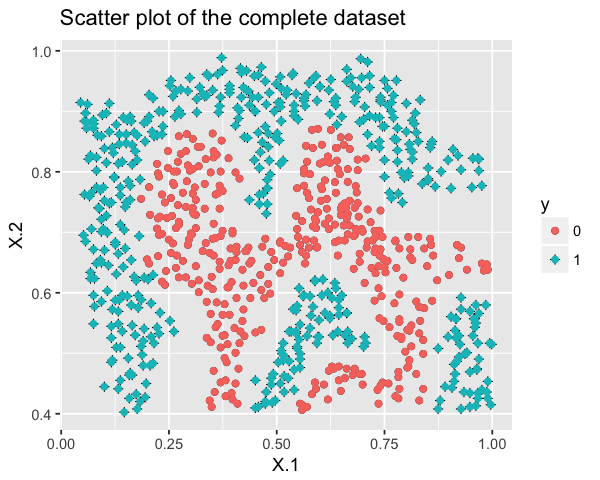In :
 # plot the training dataset
ggplot(data= train_data, aes(x=X.1, y= X.2)) + geom_point(aes(shape=y)) +geom_point(aes(color=y))+ scale_shape_manual(values=c(1,3)) + ggtitle("Scatter plot of the training dataset")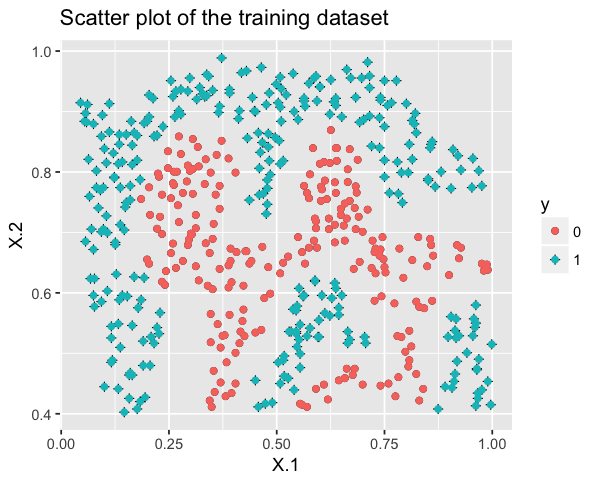In :
# Plot Validaing dataset
ggplot(data= val_data , aes(x=X.1, y= X.2)) + geom_point(aes(shape=y)) +geom_point(aes(color=y))+ scale_shape_manual(values=c(1,3)) + ggtitle("Scatter plot of the validating dataset")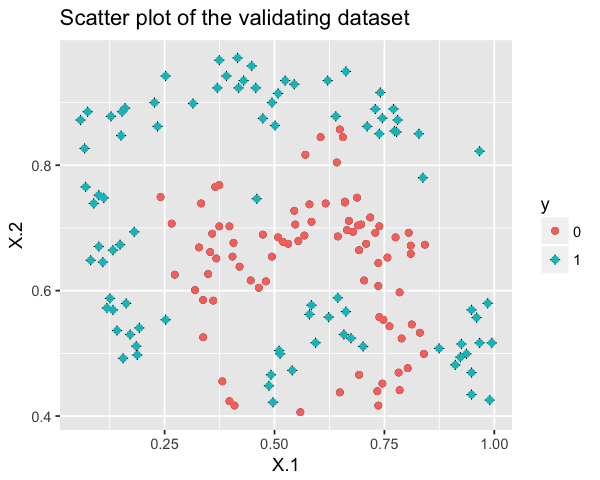In :
# plot test dataset
ggplot(data= test_data, aes(x=X.1, y= X.2)) + geom_point(aes(shape=y)) +geom_point(aes(color=y))+ scale_shape_manual(values=c(1,3)) + ggtitle("Scatter plot of the test dataset")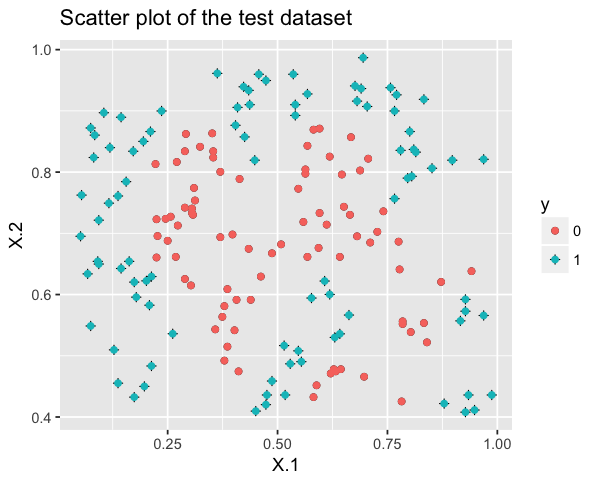#### Training dataset to traing the model¶

I used the training dataset to develop a classification model. A built in R function of svm() from “e1071” library was used to train the model. The function has two arguments, cost and gamma, to be set by the user. After making different models using training dataset with different combinations of “cost” and “gamma” values, the class lables of validating dataset was predicted. Then, the deviation of the prediction from the actual value is computed for each model. A model that has the small error for validating dataset is chosen as the best model because it has high generalizability for a new dataset.

The tune() function from “e1071” library can be applied to do the aforementioned job as follows:

In :
parm= tune(svm, train.x=train_data[,-3], train.y = as.factor(train_data$y), validation.x=val_data[,-3], validation.y = as.factor(val_data$y), range=list( gamma=c(0.01,0.03,0.1,0.3,1,3,10,30),cost=c(0.01,0.03,0.1,0.3,1,3,10,30)), tunecontrol = tune.control(sampling = "fix", fix = 1))
#summary(parm) # shows the performance


The above functions compares the performance of 64 models (Combinations of 8 gamma value and and 8 cost values of {0.01, 0.1, 0.03, 0.3, 3, 1, 10, 30}).

#### Choosing the best parameters¶

In :
# counter plot of parameters
plot(parm)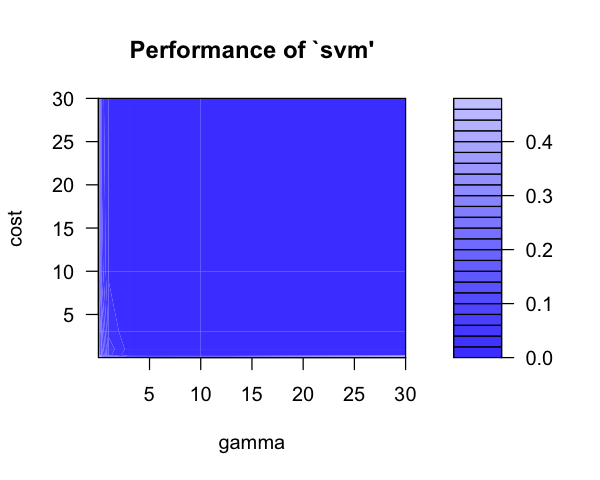In :
# 3D plot of parameters with best performance
#---------------------------------------------------------
gamma_values=unique(parm$performances$gamma)
cost_values=unique(parm$performances$cost)
persp3D(x= gamma_values , y=cost_values, z=matrix(parm$performances$error,nrow = length(gamma_values),ncol = length(cost_values))
, theta=-45, phi=0, expand=0.6,ticktype='detailed',col='blue', shade=0.75, ltheta=120, xlab="gamma",ylab="cost",zlab='Error', main='Performance of SVM for different gamma and cost values', cex.main=0.9)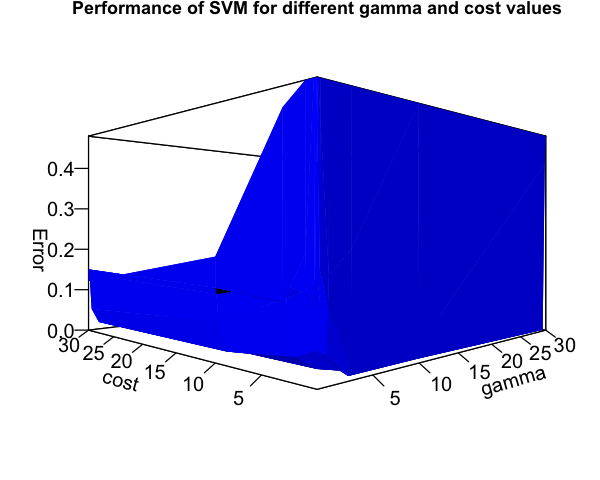The value of gamma and cost that gave the best performance for validating dataset among the 64 combinations of values are:

In :
parm$best.parameters  gammacost 3230.0 0.3 #### A model using the best parameters¶ In : # choose the best parameters and use them to make a model best_cost=parm$best.parameters$cost best_gamma=parm$best.parameters$gamma best_model= svm(y ~ . ,data =train_data, type= "C-classification", kernel= "radial", cost=best_cost , gamma=best_gamma) summary(best_model) # the summary of the best model  Call: svm(formula = y ~ ., data = train_data, type = "C-classification", kernel = "radial", cost = best_cost, gamma = best_gamma) Parameters: SVM-Type: C-classification SVM-Kernel: radial cost: 0.3 gamma: 30 Number of Support Vectors: 420 ( 195 225 ) Number of Classes: 2 Levels: 0 1  ### Plot the decision boundary¶ In : x1plot = seq(min(train_data$X.1), max(train_data$X.1), length= 100) x2plot = seq(min(train_data$X.2), max(train_data$X.2), length= 100) m = mesh(x=x1plot, y=x2plot) vals = matrix(0, nrow=nrow(m$x), ncol = ncol(m$x)) # place holder for(i in 1: ncol(m$x))
{
this_X = cbind(m$x[,i], m$y[, i])
vals[,i] = predict(best_model, this_X)

}

#-------------------------------------------------------------------
# Plot the SVM boundary
#-------------------------------------------------------------------
posw=which(mydata$y%in%1) # index for y=1 negw=which(mydata$y%in%0)  # index for y=0

plot( X.2~ X.1, data=mydata[posw,-3], col="cyan3", pch=18, main='The complete dataset with non-linear decision boundary learned by SVM',cex.main=0.8)
points( X.2 ~ X.1, data=mydata[negw,-3], col="coral1", pch=20)
legend('topright',title='y', c('0','1'),cex=1, pch=c(20,18), col=c('coral1','cyan3'), y.intersp=1.5)
par(new=TRUE) # to add the boundary line to the original data set
contour(x=m$x[,1], y=m$y[1,], z=vals, nlevels = 10, col='black', drawlabels = FALSE) # the boundary line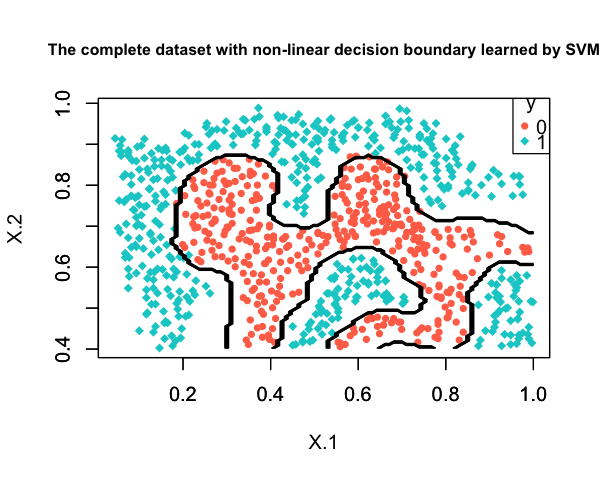### Compare the best model with some other model¶

The next plot shows two decision boundaries computed from the best model (shown in black color) and from the other model (shown in yellow color) with cost=0.1 and gamma=3. As shown in the plot, the best model predicts the class labels of y better than the other model.

In :
model2=svm(y ~ . ,data =train_data, type= "C-classification", kernel= "radial", cost=0.1 , gamma=3)

x1plot2 = seq(min(train_data$X.1), max(train_data$X.1), length= 100)
x2plot2 = seq(min(train_data$X.2), max(train_data$X.2), length= 100)
m2 = mesh(x=x1plot, y=x2plot)
vals2 = matrix(0, nrow=nrow(m2$x), ncol = ncol(m2$x)) # place holder

for(i in 1: ncol(m2$x)) { this_X2 = cbind(m2$x[,i], m2$y[, i]) vals2[,i] = predict(model2, this_X2) } #------------------------------------------------------------------- # Plot the SVM boundary #------------------------------------------------------------------- plot( X.2~ X.1, data=mydata[posw,-3], col="cyan3", pch=18, main='The complete data with non-linear decision boundary learned by SVM',cex.main=0.8) points( X.2 ~ X.1, data=mydata[negw,-3], col="coral1", pch=20) legend('topright',title='y', c('0','1'), pch=c(20,18), col=c('coral1','cyan3'), y.intersp=1.5) par(new=TRUE) # to add the boundary line to the original data set contour(x=m2$x[,1], y=m2$y[1,], z=vals2, nlevels = 10, col = "yellow", drawlabels = FALSE) # the boundary line par(new=TRUE) contour(x=m$x[,1], y=m\$y[1,], z=vals, nlevels = 10, col = "black", drawlabels = FALSE) # the boundary line